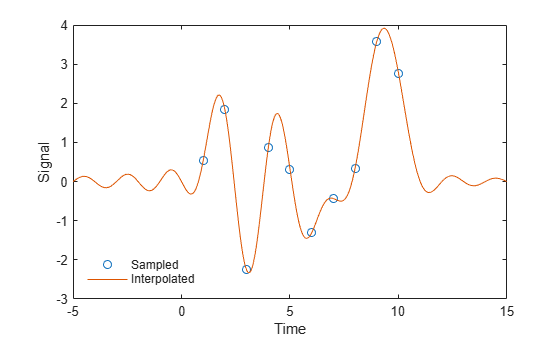# sinc

## Syntax

``y = sinc(x)``

## Description

example

````y = sinc(x)` returns an array, `y`, whose elements are the sinc of the elements of the input, `x`. The output `y` is the same size as `x`.```

## Examples

collapse all

Perform ideal bandlimited interpolation of a random signal sampled at integer spacings.

Assume that the signal to interpolate, `x`, is 0 outside of the given time interval and has been sampled at the Nyquist frequency. Reset the random number generator for reproducibility.

```rng default t = 1:10; x = randn(size(t))'; ts = linspace(-5,15,600); [Ts,T] = ndgrid(ts,t); y = sinc(Ts - T)*x; plot(t,x,'o',ts,y) xlabel Time, ylabel Signal legend('Sampled','Interpolated','Location','SouthWest') legend boxoff```## Input Arguments

collapse all

Input array, specified as a real-valued or complex-valued scalar, vector, matrix, N-D array, or `gpuArray` object. When `x` is nonscalar, `sinc` is an element-wise operation.

See Run MATLAB Functions on a GPU (Parallel Computing Toolbox) and GPU Support by Release (Parallel Computing Toolbox) for details on `gpuArray` objects.

Data Types: `single` | `double`
Complex Number Support: Yes

## Output Arguments

collapse all

Sinc of the input array, `x`, returned as a real-valued or complex-valued scalar, vector, matrix, N-D array, or `gpuArray` object of the same size as `x`.

collapse all

### sinc

The sinc function is defined by

`$\mathrm{sinc}t=\left\{\begin{array}{cc}\frac{\mathrm{sin}\pi t}{\pi t}& t\ne 0,\\ 1& t=0.\end{array}$`

This analytic expression corresponds to the continuous inverse Fourier transform of a rectangular pulse of width 2π and height 1:

`$\mathrm{sinc}t=\frac{1}{2\pi }\text{\hspace{0.17em}}\text{\hspace{0.17em}}{\int }_{-\pi }^{\pi }{e}^{j\omega t}\text{\hspace{0.17em}}d\omega .$`

The space of functions bandlimited in the frequency range $\omega =\left(-\pi ,\pi \right]$ is spanned by the countably infinite set of sinc functions shifted by integers. Thus, you can reconstruct any such bandlimited function g(t) from its samples at integer spacings:

`$g\left(t\right)=\sum _{n=-\infty }^{\infty }g\left(n\right)\mathrm{sinc}\left(t-n\right).$`